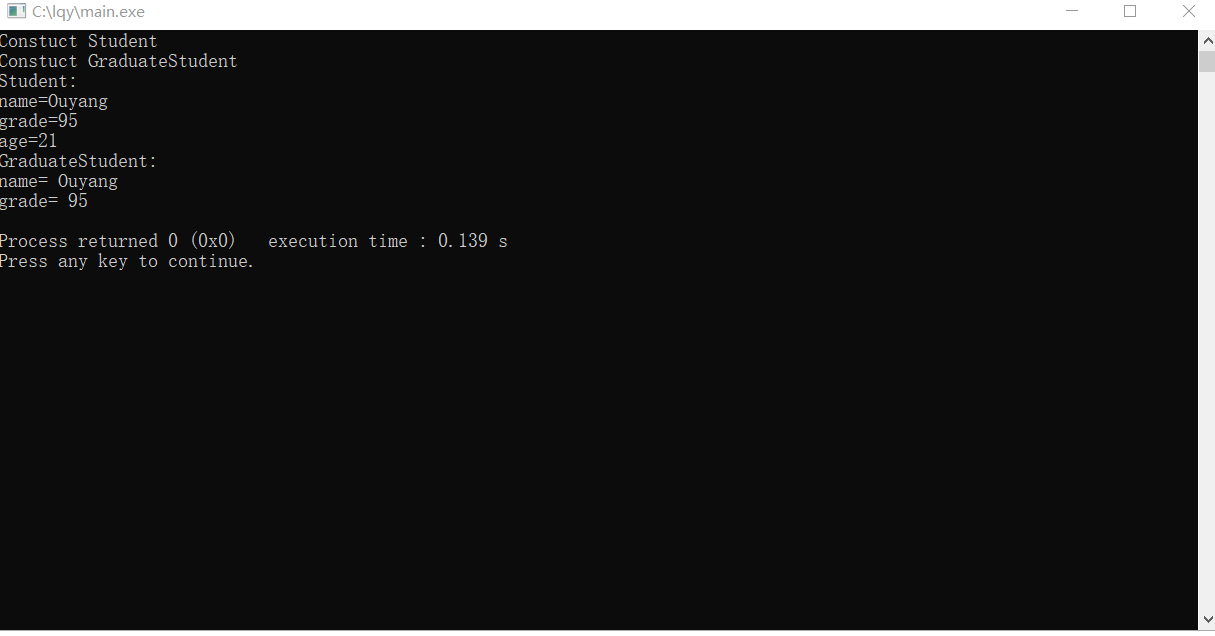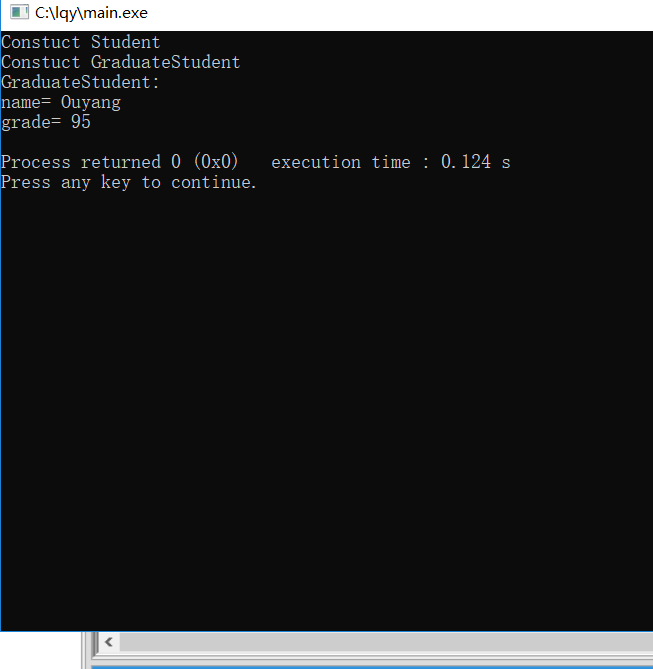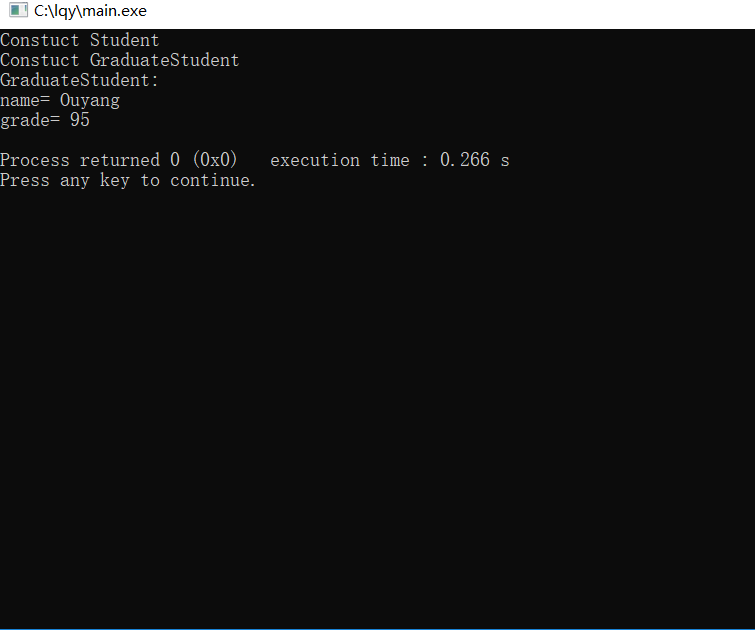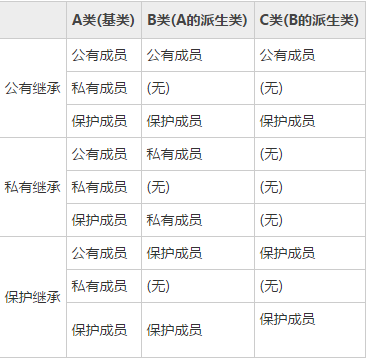# C++作业

1、继承的基本概念：

继承是c++语言一个重要的机制，该机制自动地为一个类提供来自另一个类的操作和数据结构，这使得程序员只需在新类中定义已有的类中没有的成分来建立一个新类。

继承有什么特点：

（1）、子类拥有父类的所有属性和行为
（2）、子类也是一种特殊的父类
（3）、子类对象可以当父类对象使用
（4）、子类中可以添加父类没有的属性和方法
（5）、子类对象可以直接初始化为父类对象
（7）、继承是面向对象编程中代码复用的重要手段

2、继承的分类详解及访问权限：

1.公有继承：

格式：

class <派生类名>: public 基类名1>,public<基类名2>,…
{
<派生类类体>
};

访问权限：当一个类派生自公有基类时，基类的公有成员也是派生类的公有成员，基类的保护成员也是派生类的保护成员，基类的私有成员不能直接被派生类

代码解释：

#include<iostream>
#include<string>
using namespace std;
class Student
{
public :
Student(string s, int g,int a)
{
cout << "Constuct Student" << endl;
name = s;
age = a;
}
void print()
{
cout << "Student:" << endl;
cout << "name=" << name << endl;
cout << "age=" << age << endl;
}
protected:
string name;
private:
int age;
};

class GraduateStudent :public Student                        //继承方式i
{
public:
GraduateStudent(string s, int g, int a) :Student(s, g, a)
{
cout << "Constuct GraduateStudent" << endl;
}
/*
公有继承方式，会把基类的公有成员（变量和函数）继承到子类公有成员，保护成员
变成基类的保护成员，但是私有成员子类也一样不可以访问
*/
void print1()
{
cout << "GraduateStudent:" << endl;
cout << "name= " << name << endl;
cout << "grade= " << grade << endl;
}

};

void main()
{
GraduateStudent g("Ouyang", 95, 21);
g.print();                     //子类可以直接访问基类公共成员成员
g.print1();
system("pause");
}2.保护继承：

格式：

class <派生类名>: private基类名1>,private<基类名2>,…
{
<派生类类体>
};

访问权限：基类公有成员，子类中继承为自己的保护成员，在派生类可以访问，在外部不可以访问。基类保护成员，子类中继承为自己的保护成员，在派生类可以访问，在外部不可以访问。基类私有成员，子类一样不可以访问基类的私有成员。

代码解释：

#include<iostream>
#include<string>
using namespace std;
class Student
{
public :
Student(string s, int g,int a)
{
cout << "Constuct Student" << endl;
name = s;
age = a;
}
void print()
{
cout << "Student:" << endl;
cout << "name=" << name << endl;
cout << "age=" << age << endl;
}

string name;   //公有成员
private:
int age;
};

class GraduateStudent :protected Student                        //继承方式i
{
public:
GraduateStudent(string s, int g, int a) :Student(s, g, a)   {//调用基类的构造函数，构造基类
cout << "Constuct GraduateStudent" << endl;
}
/*
保护继承方式，会把基类的公有成员或者保护成员（变量和函数）变成子类的保护成员，但是私有成员子类也一样不可以访问
*/
void print1(){
cout << "GraduateStudent:" << endl;
cout << "name= " << name << endl;
cout << "grade= " << grade << endl;
}
};int main()
{
GraduateStudent g("Ouyang", 95, 21);
//g.print();   编译出错                  无法直接访问子类的保护成员了
g.print1();
return 0;
}3.私有继承：

格式：

class <派生类名>: private基类名1>,private<基类名2>,…
{
<派生类类体>
};

访问权限： 基类公有成员，子类中继承为自己的私有成员，在派生类可以访问，在外部不可以访问。 基类保护成员，子类中继承为自己的私有成员，在派生类可以访问，在外部不可以访问。 基类私有成员，子类一样不可以访问基类的私有成员。

代码解释：

#include<iostream>
#include<string>
using namespace std;
class Student
{
public :
Student(string s, int g,int a)
{
cout << "Constuct Student" << endl;
name = s;
age = a;
}
void print()
{
cout << "Student:" << endl;
cout << "name=" << name << endl;
cout << "age=" << age << endl;
}
string name;   //公有成员
private:
int age;
};

class GraduateStudent :private Student                        //继承方式i
{
public:

GraduateStudent(string s, int g, int a) :Student(s, g, a)   //调用基类的构造函数，构造基类
{
cout << "Constuct GraduateStudent" << endl;
}
/*
保护继承方式，会把基类的公有成员或者保护成员（变量和函数）变成子类的保护成员，但是私有成员子类也一样不可以访问
*/
void print1()
{
cout << "GraduateStudent:" << endl;
cout << "name= " << name << endl;
cout << "grade= " << grade << endl;
}
};

int main()
{
GraduateStudent g("Ouyang", 95, 21);
//g.print();   //编译出错无法直接访问子类的私有成员了
g.print1();
return 0;
}：

运行结果：posted @ 2019-10-13 14:57  ****l****  阅读(171)  评论(0编辑  收藏  举报